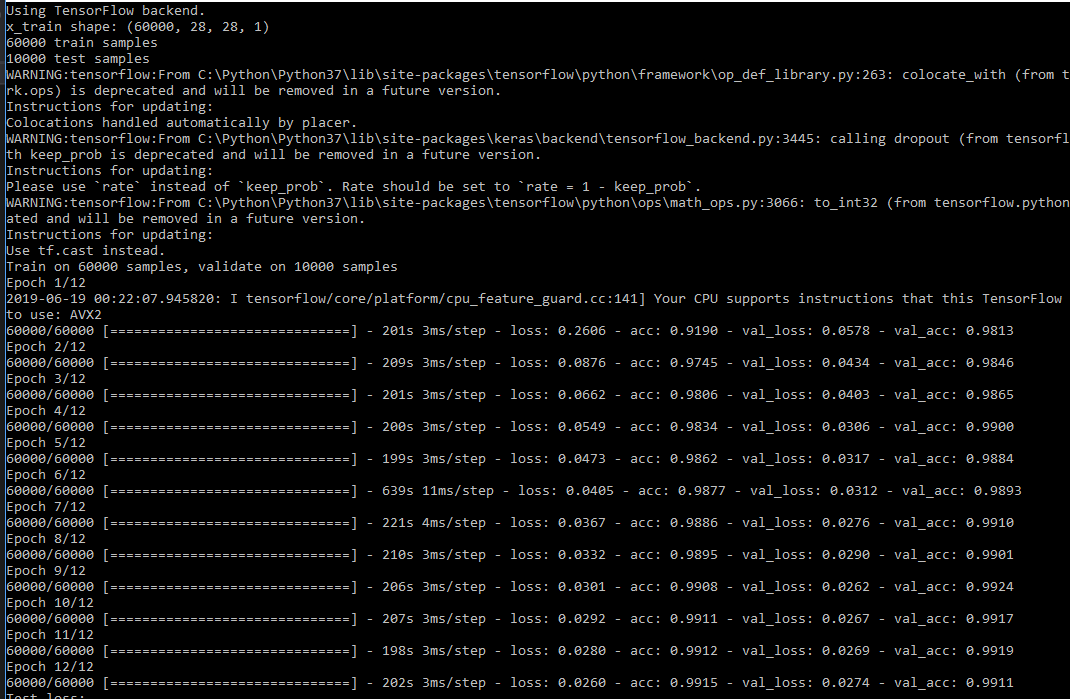{{ message }}

# SciSharp / Keras.NET Public

Keras.NET is a high-level neural networks API for C# and F#, with Python Binding and capable of running on top of TensorFlow, CNTK, or Theano.

Switch branches/tags
Nothing to show

## Files

Failed to load latest commit information.
Type
Name
Commit time
Aug 13, 2020
Jun 23, 2019
Sep 19, 2021
Sep 19, 2021
Jun 26, 2019
Jun 16, 2019
Sep 19, 2021
Aug 21, 2020

Keras.NET is a high-level neural networks API for C# and F# via a Python binding and capable of running on top of TensorFlow, CNTK, or Theano. It was developed with a focus on enabling fast experimentation. Being able to go from idea to result with the least possible delay is key to doing good research.

Use Keras if you need a deep learning library that:

• Allows for easy and fast prototyping (through user friendliness, modularity, and extensibility).

• Supports both convolutional networks and recurrent networks, as well as combinations of the two.

• Runs seamlessly on CPU and GPU.

## Prerequisite

• Install keras, numpy and one of the backends (Tensorflow/CNTK/Theano). Keras is now bundled with Tensorflow 2.0, so the easiest way to install Keras and Tensorflow at the same time is to simply install Tensorflow 2.0.

## Nuget

Install from nuget: https://www.nuget.org/packages/Keras.NET

``````dotnet add package Keras.NET
``````

## Example with XOR sample (C#)

```//Load train data
NDarray x = np.array(new float[,] { { 0, 0 }, { 0, 1 }, { 1, 0 }, { 1, 1 } });
NDarray y = np.array(new float[] { 0, 1, 1, 0 });

//Build sequential model
var model = new Sequential();
model.Add(new Dense(32, activation: "relu", input_shape: new Shape(2)));

//Compile and train
model.Compile(optimizer:"sgd", loss:"binary_crossentropy", metrics: new string[] { "accuracy" });
model.Fit(x, y, batch_size: 2, epochs: 1000, verbose: 1);

//Save model and weights
string json = model.ToJson();
File.WriteAllText("model.json", json);
model.SaveWeight("model.h5");

Output:## MNIST CNN Example (C#)

Python example taken from: https://keras.io/examples/mnist_cnn/

```int batch_size = 128;
int num_classes = 10;
int epochs = 12;

// input image dimensions
int img_rows = 28, img_cols = 28;

Shape input_shape = null;

// the data, split between train and test sets
var ((x_train, y_train), (x_test, y_test)) = MNIST.LoadData();

if(Backend.ImageDataFormat() == "channels_first")
{
x_train = x_train.reshape(x_train.shape, 1, img_rows, img_cols);
x_test = x_test.reshape(x_test.shape, 1, img_rows, img_cols);
input_shape = (1, img_rows, img_cols);
}
else
{
x_train = x_train.reshape(x_train.shape, img_rows, img_cols, 1);
x_test = x_test.reshape(x_test.shape, img_rows, img_cols, 1);
input_shape = (img_rows, img_cols, 1);
}

x_train = x_train.astype(np.float32);
x_test = x_test.astype(np.float32);
x_train /= 255;
x_test /= 255;
Console.WriteLine(\$"x_train shape: {x_train.shape}");
Console.WriteLine(\$"{x_train.shape} train samples");
Console.WriteLine(\$"{x_test.shape} test samples");

// convert class vectors to binary class matrices
y_train = Util.ToCategorical(y_train, num_classes);
y_test = Util.ToCategorical(y_test, num_classes);

// Build CNN model
var model = new Sequential();
activation: "relu",
input_shape: input_shape));
model.Add(new Conv2D(64, (3, 3).ToTuple(), activation: "relu"));

model.Compile(loss: "categorical_crossentropy",

model.Fit(x_train, y_train,
batch_size: batch_size,
epochs: epochs,
verbose: 1,
validation_data: new NDarray[] { x_test, y_test });
var score = model.Evaluate(x_test, y_test, verbose: 0);
Console.WriteLine(\$"Test loss: {score}");
Console.WriteLine(\$"Test accuracy: {score}");```

Output

Reached 98% accuracy within 3 epoches.# Documentation

https://scisharp.github.io/Keras.NET/Keras.NET is a high-level neural networks API for C# and F#, with Python Binding and capable of running on top of TensorFlow, CNTK, or Theano.